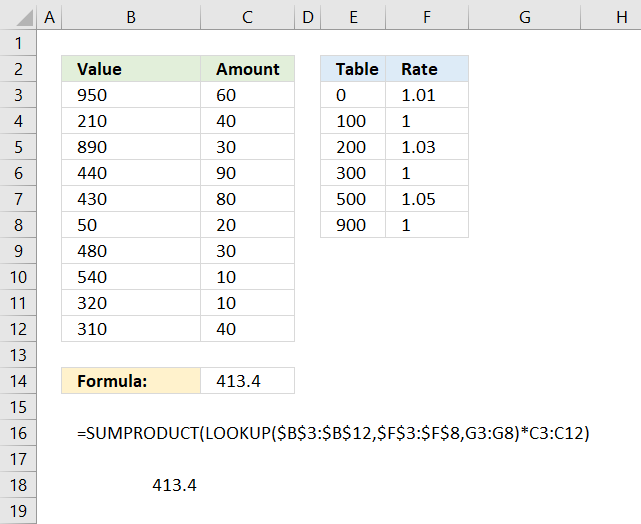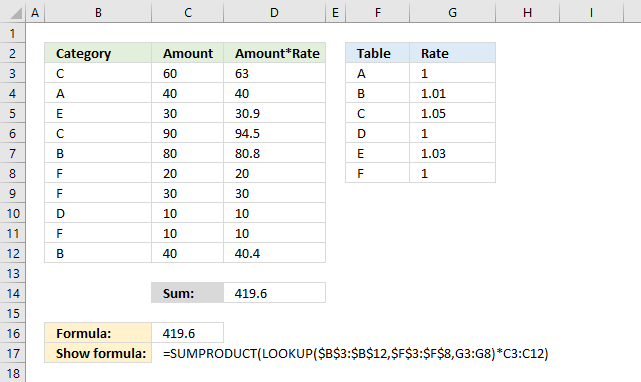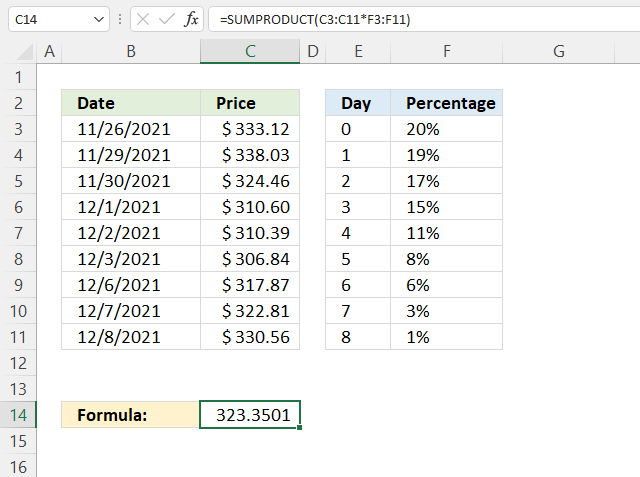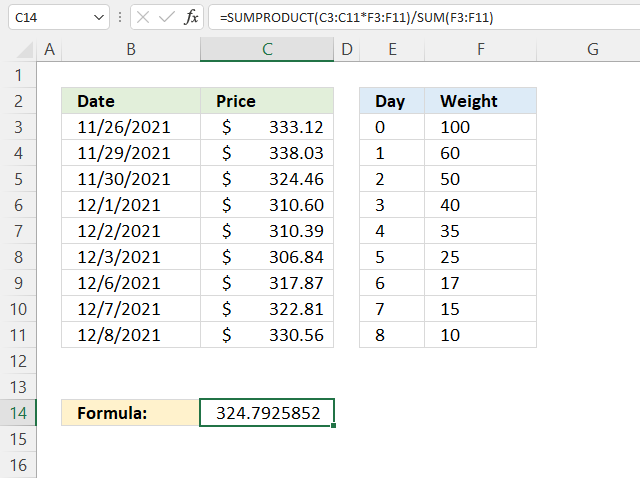Author: Oscar Cronquist Article last updated on January 08, 2023I have demonstrated in a previous post how to simplify nested IF functions, in this article I will show you how to simplify your SUMPRODUCT formulas regarding multiple criteria.

## 1. SUMPRODUCT - nested IF functions

The formula in cell C14 multiplies numbers with a rate based on the size of the number and returns a total. The table in E2:F8 shows the different rates and the corresponding criteria.

For example, numbers between 0 (zero) and 100 have a rate multiplier of 1.01. Numbers between 100 and 200 are multiplied by 1.

Formula in cell C14:

=SUMPRODUCT(LOOKUP(\$B\$3:\$B\$12,\$E\$3:\$E\$8,F3:F8)*C3:C12)

### 1.1 Explaining formula

In most cases, there is no need for IF functions in SUMPRODUCT formulas, this is true in this case as well, the criteria below are complicated to build with IF functions.

0 <= value < 100 Rate: 1.01
100 <= value < 200 Rate: 1
200 <= value < 300 Rate: 1.03
300 <= value < 500 Rate: 1
500 <= value < 900 Rate: 1.05
900 <= value Rate: 1

#### Step 1 - Map numbers to corresponding rates

If a value in column B is matching one of the above ranges the corresponding rate is used.

However, it can be easily simplified using the LOOKUP function. The following formula is entered in cell C14 in the image above.

A small table is easy to build, shown in columns E and F. The LOOKUP function requires the values in E3:E8 to be sorted in ascending order for it to work properly.

Instead of using one lookup value in the first argument, I am using an entire cell range.

LOOKUP(B3:B12,E3:E8,F3:F8)

The rate is determined by the value in B3:B12.

LOOKUP({950; 210; 890; 440; 430; 50; 480; 540; 320; 310},{0; 100; 200; 300; 500; 900},{1.01; 1; 1.03; 1; 1.05; 1})

The LOOKUP function matches the values in B3:B12 to the values in F3:F8 and returns the corresponding value from G3:G8 simultaneously.

{1; 1.03; 1.05; 1; 1; 1.01; 1; 1.05; 1; 1}

#### Step 2 - Multiply numbers with rates

Now we know which rates to use, it is now possible to multiply the amounts.

LOOKUP(\$B\$3:\$B\$12,\$E\$3:\$E\$8,F3:F8)*C3:C12

becomes

{1; 1.03; 1.05; 1; 1; 1.01; 1; 1.05; 1; 1}*C3:C12

becomes

{1; 1.03; 1.05; 1; 1; 1.01; 1; 1.05; 1; 1}*{60; 40; 30; 90; 80; 20; 30; 10; 10; 40}

and returns

{60; 41.2; 31.5; 90; 80; 20.2; 30; 10.5; 10;40}.

#### Step 3 - Add results and return a total

Lastly, the SUMPRODUCT function adds all numbers and returns a total.

SUMPRODUCT({60; 41.2; 31.5; 90; 80; 20.2; 30; 10.5; 10; 40})

and returns 413.4 in cell C14.

#### Equivalent formula using nested IF functions

So what would the equivalent formula look like using IF functions?

=SUMPRODUCT(IF((B3:B12>=0)*(B3:B12<100), 1.01, IF((B3:B12>=200)*(B3:B12<300), 1.03, IF((B3:B12>=500)*(B3:B12<900), 1.05, 1)))*C3:C12)

#### Verify that the formula worksColumn D is a column to verify the calculation, you don't need it.

## 2. SUMPRODUCT - weighted average based on percentagesThe image above demonstrates a formula that calculates the weighted average for a given date using percentages. This technique can be used to create a weighted moving average often used in stock charts.

The weighted moving average puts more weight towards recent dates making the weighted moving average follow price more closely than the simple moving average. The image above, however, puts more weight on earlier dates.

Formula in cell C14:

=SUMPRODUCT(C3:C11*F3:F11)

The percentages are specified in F3:F11 and the total must be 1. 0.2 + +.19 + 0.17 + 0.15 + 0.11 + 0.08 + 0.06 + 0.03 + 0.01 equals 1.

To create a moving weighted average lock the reference pointing to the percentages by converting it to an absolute cell reference.

=SUMPRODUCT(C3:C11*\$F\$3:\$F\$11)

You can now copy cell C14 and paste it to cells below to calculate a running weighted average or weighted moving average. You need to have more price data than I have shown in the image above to make it work.

### Explaining formula in cell C14

#### Step 1 - Multiply price data with percentages

The asterisk lets you multiply value with value, this works fine with cell ranges as well.

C3:C11*\$F\$3:\$F\$11

becomes

{333.12; 338.03; 324.46; 310.6; 310.39; 306.84; 317.87; 322.81; 330.56}*{0.2; 0.19; 0.17; 0.15; 0.11; 0.08; 0.06; 0.03; 0.01}

and returns

{66.624; 64.2257; 55.1582; 46.59; 34.1429; 24.5472; 19.0722; 9.6843; 3.3056}.

#### Step 2 - Add results and return a total

SUMPRODUCT(C3:C11*F3:F11)

becomes

{66.624; 64.2257; 55.1582; 46.59; 34.1429; 24.5472; 19.0722; 9.6843; 3.3056}

and returns 323.3501 in cell C14.

## 3. SUMPRODUCT - weighted averageThe example demonstrates how to use weights to create a weighted average, there is no requirement that the sum is 100 or 1 the formula takes care of that.

Formula in cell C14:

=SUMPRODUCT(C3:C11*F3:F11)/SUM(F3:F11)

### Explaining formula in cell C14

#### Step 1 - Multiply price data with weights

The asterisk lets you multiply value with value in Excel, this works fine with cell ranges as well.

C3:C11*F3:F11

becomes

{333.12; 338.03; 324.46; 310.6; 310.39; 306.84; 317.87; 322.81; 330.56}*{100; 60; 50; 40; 35; 25; 17; 15; 10}

and returns

{33312; 20281.8; 16223; 12424; 10863.65; 7671; 5403.79; 4842.15; 3305.6}.

#### Step 2 - Add results and return a total

SUMPRODUCT(C3:C11*F3:F11)

becomes

SUMPRODUCT({33312; 20281.8; 16223; 12424; 10863.65; 7671; 5403.79; 4842.15; 3305.6})

and returns 114326.99.

#### Step 3 - Divide by sum

The SUM function adds numbers from a cell range and returns a total.

SUMPRODUCT(C3:C11*F3:F11)/SUM(F3:F11)

becomes

114326.99/SUM(F3:F11)

becomes

114326.99/352

and returns 324.792585227273.